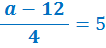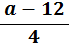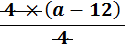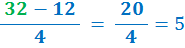### Solving Equations − 2

Here we use a combination of Solving Equations − Add and Subtract and Solving Equations − Multiply and Divide. Please look at the above before continuing

• #### Example 1.   3(6 + b) = 15   What is the value of b?

•  i. Expand by multiplying out the brackets on the left hand side (LHS) of the equation

 3(6 + b) = 15 18 + 3b = 15

 ii. Subtract 18 from both sides

 18 + 3b = 15 − 18 = − 18
 3b = −  3

 iii. Divide both sides by 3

 3b = −  3 ÷ 3 = ÷  3
 b = − 1

 iv. To verify, put the value of b = − 1 back into the original equation:

3(6 + (−1)) = 3(6 − 1) = 3(5) = 15

• #### Example 2.

•What is the value of a?

 i. The inverse of divide by 4 is multiply by 4. So multiply both sides by 4= 5 × 4 = × 4= 20
 a − 12 = 20

 ii. The inverse of − 12 is + 12. So add 12 to both sides

 a − 12 = 20 + 12 = +12
 a = 32

 iii. To check, put the value of a = 32 back into the original equation:• #### Example 3.   40 + 2d = 5d − 20   What is the value of d?

•  i. The inverse of − 20 is + 20. So add 20 to both sides

 40 + 2d = 5d − 20 + 20 = + 20
 60 + 2d = 5d

 ii. Move variable d to one side so inverse of + 2d is − 2d. So subtract 2d from both sides

 60 + 2d = 5d − 2d = − 2d
 60 = 3d

 iii. Divide both sides by 3

 60 = 3d ÷ 3 = ÷ 3
 20 = d

 iv. To verify, put the value of d = 20 back into the original equation:

both sides are equal

#### Note: 'what is the value of y' is the same as 'solve y'to: